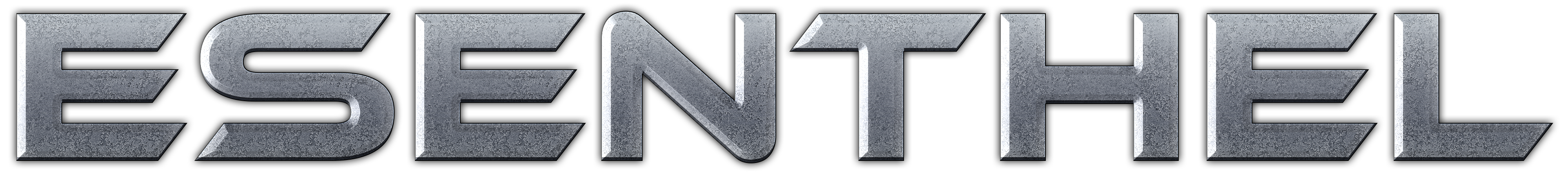About Store Forum Documentation ContactInvalid variable value Author Message
neuroliquidMember

 Post: #1 Invalid variable value I don't understand why this app gives output other than 0. Compiled and tested on 2 different linux. I am not sure if this is my misunderstanding of c++ or some kind of bug. Can anyone elucidate what is going on there? Code: ```class A { public:       class B    {    public:          B(int f){}    };       B b=0;       A(bool loop){} }; class O : public A { public:    int problem=0;    using A::A; }; class Z { public:    O o(true);    Z()    {       Log(S+o.problem+'\n');       Exit();    } }; void InitPre() {    EE_INIT();    App.flag=APP_HIDDEN; } bool Init() {    Z *z=new Z;    return true; } void Shut(){} bool Update(){} void Draw(){}```
05-16-2018 02:09 PM
HougeMemberPost: #2 RE: Invalid variable value You call "O o(true);" constructor, it's from A class, not from O, so "problem" is not initialized. If you use this: Code: ```class Z { public:    O o;//(true);    Z()    {    ...``` It will be OK. (This post was last modified: 05-16-2018 04:38 PM by Houge.)
05-16-2018 04:38 PM
EsenthelPost: #3 RE: Invalid variable value I would write the code this way: Code: ```class A {    class B    {       B(int f){}    };       B b=0;       A(bool loop){} }; class O : A {    int problem=0;    O(bool loop) : A(loop) {} }; class Z {    O o(true);    Z()    {       Exit(o.problem);    } }; void InitPre() {    EE_INIT();    Z z;    App.flag=APP_EXIT_IMMEDIATELY; } bool Init() {    return false; } void Shut(){} bool Update(){return false;} void Draw(){}``` BTW. In EE Code Editor 'class' is already public.